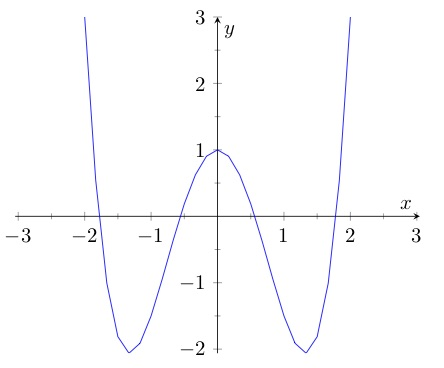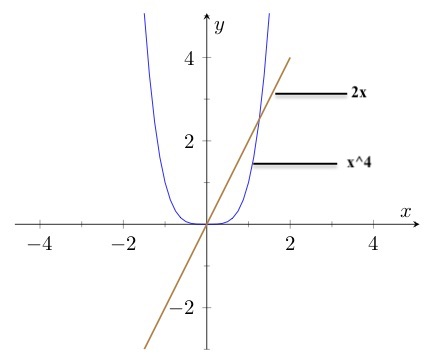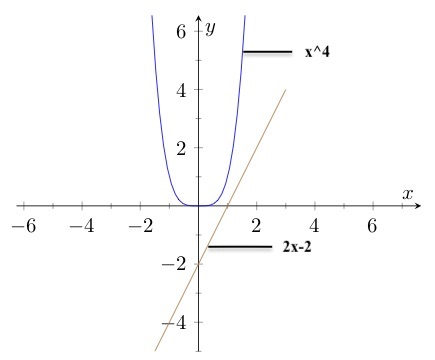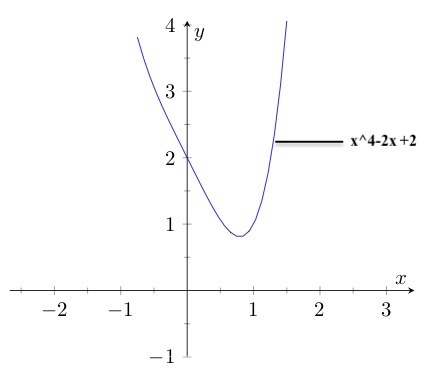## Finding a Second Degree Equation from Its Solutions

This post about finding a second degree equation from its solutions is part of a series of posts to help you prepare for the Advanced Algebra and Functions part of the Accuplacer test.

## Question

x = 4 is the only solution to one of the equations below.

Which one?

A.

B.

C.

D.

## Solution

### Note

For solving equations, the terms “root,” “solution,” “zero,” and “x-intercept” all mean pretty much the same thing, an answer to the problem. “Zero” and “x-intercept” come from the way equations are usually graphed for a problem: The equation is set equal to zero and then solved for the x-value that makes it all equal zero. On a graph, that x-value is an x-intercept.

### Back to the Question

The factor theorem says that if   is a solution to , then  must be a factor of . For example, if is a solution,  must be a factor of .

For which of the answer choices is  a factor? Choices B and D. What’s the right answer?

The other clue in the question is that  is the only solution. The factor theorem says also that if  is a factor of , then c must be a solution to . Note that the equation in answer choice D also contains another factor: . If   is a factor, then -4 is a solution. That would make  a second solution — but the question says there can be only one, so D cannot be the correct answer.

That means the correct answer is choice B.

The Accuplacer sample problem on which this one is based is #12 in the sample questions for the Accuplacer Advanced Algebra and Functions test.

# How Many x-Intercepts?

## Question

What is the maximum number of zeros (also called x-intercepts) the function    may have? What is the minimum? c, a constant, may be any real number.

## Solution

The maximum number zeros of a polynomial function is equal to the function’s degree. The function  is fourth degree, so it may have up to four zeros. A fourth-degree function may look like this:Four is the max. Will the function  necessarily have four? Let’s try to narrow down the possibilities.

Try a graph. You can graph this function if you assign a value to c. Let’s try , so that the function is . That graphs up like this:That gives you two x-intercepts. but with a different value of c could this function may have more x-intercepts — or fewer?

At the x-intercepts, the y-value is 0:

That means

That means that for this function, at the zeros, the fourth-degree monomial   equals the linear function . Let’s graph these two pieces separately for  , the value of c that we’ve seen gives two x-intercepts.These two pieces intersect in two placesone for each of the two zeros. Are any other points of intersection possible? On the left-hand side, the fourth-degree term just keeps going up while the line just keeps going down, so there are no more points of intersection on the left. On the right-hand side to the right of both points of intersection, the fourth-degree term is always steeper than the line and is therefore always greater, so there are no more points of intersection on the right. Remember that each point of intersection on this graph corresponds to a zero on the function , so that means no other zero is possible for the function.

But that doesn’t cover all possibilities, because we considered only one value for c. What if c takes a different value? Then the line slides up or down. If  , the line slides up and there will two points of intersection, one at which x is less than zero and one in which x is greater than zero.

Slide it down just a little from and there are still two points of intersection, this time both positive. Slide it farther and eventually it will not intersect the fourth-degree curve at all.For even higher values of c – meaning the line slides farther down on the y-axis – the function has no zeros.

So for the function , the minimum number of x-intercepts is zero and the maximum number is two.